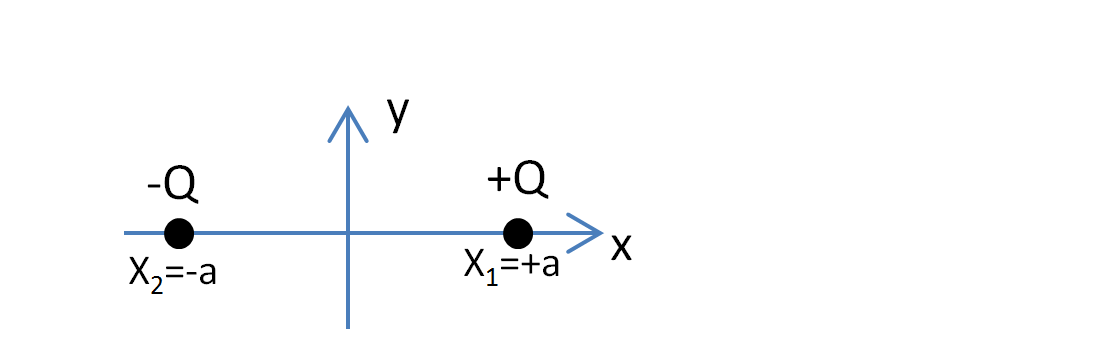# Problem: Two charged particles are located at x = -a and x = +a, as shown below, on the x-axis. The electric field produced by these two charges at any point on the y-axis, is always A) in the +y direction B) in the -y direction C) in the +x direction D) in the -x direction

###### FREE Expert Solution
88% (248 ratings)
###### Problem Details

Two charged particles are located at x = -a and x = +a, as shown below, on the x-axis. The electric field produced by these two charges at any point on the y-axis, is always

A) in the +y direction

B) in the -y direction

C) in the +x direction

D) in the -x direction